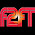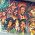## Wednesday, June 24, 2015

### 14 54 61 105 | The Gyrocopter Landing of April 15, 2015

Recall 61-year old 'Doug Hughes' landed a 'Gyrocopter' on the White House lawn on April 15, 2015- the anniversary of the death of Lincoln and the Titanic fully submerging.
• 61-years old
• Gyrocopter = 7+7+9+6+3+6+7+2+5+9 = 61 (Gyrocopter & President Obama = 142 in SE)
• Doug Hughes = 4+6+3+7+8+3+7+8+5+1 = 52/61
• Obama was born in '61
• South Carolina hung Confederate Flag in the year 1961...
• Major debate right now nationally about Confederate Flag
• Based on a 'Church Shooting'
• Church = 3+8+21+18+3+8 = 61
Making matters even more interesting, this incident came 61-days after Lupercalia, which all the major hoaxes have been measured by.

February 13 is the 44th day of the year, the date of Lupercalia.
• Lupercalia = 3+3+7+5+9+3+1+3+9+1 = 44
• Lupercalia on the 44th day of the year
This date is also 161 days from the Pope's visit.
• Pope = 16+15+16+5 = 52
• Doug Hughes = 4+6+3+7+8+3+7+8+5+1 = 52/61

With regards to the Titanic-
It should be noted that Futility, Wreck of the Titan was published January 1, 1898, 14-years before the Titanic sunk . (Dead = 14)(End = 14)(It has been 14-years since 2001, come this September.)
• September = 1+5+7+2+5+4+2+5+9 = 40/49
• Twin Towers = 2+5+9+5+2+6+5+5+9+1 = 49/58
• Iceberg = 9+3+5+2+5+18+7 = 49
• The towers were proposed by David Rockefeller, and approved in part by 49th Governor Nelson Rockefeller.  The name Rockefeller in Simple English Gematria
The book is about the largest ship ever built, traveling from Mainland UK to the United States, thought to be an unthinkable ship.  On its first sail, it hits an iceberg, sinking to the bottom of the ocean, the beginning and quick end of the TITAN.

What letters are missing from this "real life story"?

TitanIC.  I and C, 9 and 3.
• IC = 93
• Thelema worships 93.
• United States of America = 93 (With States exception on Big S)
• 1776 = 17+76 = 93
• Propaganda = 16+18+15+16+1+7+1+14+4+1 = 93
• Many important people in history have '93 names' in Gematria
• Malcolm X
• Martin Luther King Jr.
• Rosa Louise Mccaulley Parks
• Bob Marley
• Beyonce Giselle Knowles
• Michael Anthony Nutter
• More... and of all races... and yes, many, many more...
• Very important number to founding of nation, and especially NY State
• The New York Times = 93
• One World Trade Center = 93
• '93 WTC Bombing
• Flight 93 of 33 passengers
• Plane 11 hit floors 93 through 99
• UN is 39 floors tall
• NY established = 7/26/1788 = 7+2+6+1+7+8+8 = 39
• NY = 14+25 = 39
• New York = 5+5+5+7+6+9+2 = 39 (Pytahgorean, reducing)
• Empire = 5+4+7+9+9+5 = 39
Of course, all of these numbers trade back to Judaism and the Old Testament of 39 Books.  What's that 'racist' nickname for New York?  Now you get it, with the numbers.98, that number tied to Lupercalia, from death and destruction to pro sports 1/1/1898 = 1+1+18+98 = 118 Just Do It = 118
And the author's name is also a major clue.
• Morgan= 4+6+9+7+1+5 = 32
• Robertson = 9+6+2+5+9+2+1+6+5 = 45/54
• Morgan Robertson = 77/86
• Judaism = 77
• The American Meridian is the 77th Merdian
With regards to Lincoln-
Lincoln died on April 15, 1865, the same day the Gyrocopter landed.
• 4/15/65 = 4+15+65 = 84
• Obama's birthday is 8/4
• Obama = 84 in Jewish Gematria (50+2+1+30+1 = 84)
• United States of America = 84 (Pythagorean)
• Orwell's Book... 1984
Also, think about how Obama has connections to Illinois, the "Land of Lincoln., the 'LOL'.  I wonder just how "honest" Abe really was, thinking back to how he was shot in Baptist Church, built in 1833, by an actor.
• Baptist = 2+1+7+2+9+1+2 = 24/33
• Built in '33
Last year, on Obama's birthday, August 4, 2014, the 9th of Av, James Brady was shot and killed.  James Brady is the same man who is known for saving Ronald Reagan from an assassin.  Ronald Reagan was the 33rd Governor of California and a former actor.
• Ronald = 18+15+14+1+12+4 = 64
• Reagan = 18+5+1+7+1+14 = 46
• Ronald Reagan = 110
• President = 16+18+5+19+9+4+5+14+20 = 110
Of course, all of this is architected by Masonic Zionists, or Freemasons.
• Freemason = 6+18+5+5+13+1+19+15+14 = 96
Do you recall the date of the JFK assassination?
• 11/22/63 = 11+22+63 = 96
• 11/22/1963 = 11+22+19+63 = 115 (Freemasons = 115; Killing = 115 JG)

1.Zach, Here is an article you should like with interesting dates and titles. It claims that George Washington laid a foundation stone for DC at 3:30 PM on April 15, 1791. That is 74 years before Lincoln died or 488 minutes or 8 hours and 8 minutes short of exactly 74 years. Lincoln was pronounced dead at 7:22 AM.

http://www.abeldanger.net/2012/12/washington-dc-rome-on-potomac-jesuits.html

1.Nice work, I actually wrote about that somewhere in my earliest posts.

2.From 4/15 to 9/9 is 147 days, or 21 weeks

3.One note Zach
In the movie Long Kiss Goodnight
The white guy holding Samuel L. Jackson hostage tells him we are going to knock down the Twin Towers and blame it on the Muslims.
Then Jackson ask what for, he says to increase our defense spending...
Hmmm SMDH

4.With regards to the number 118, Pope Francis is sometimes called HOLY FATHER = 678 / 708 / 118 (55). He is also referenced as HIS HOLINESS = 419 / 822 / 137 (56).